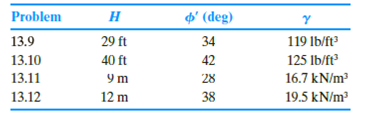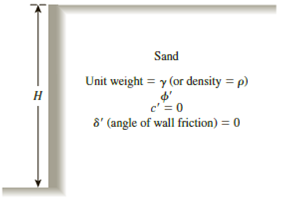Chapter 13, Problem 13.11PPrinciples of Geotechnical Enginee...

9th Edition
Braja M. Das + 1 other
ISBN: 9781305970939

Solutions

Chapter
SectionPrinciples of Geotechnical Enginee...

9th Edition
Braja M. Das + 1 other
ISBN: 9781305970939
Textbook Problem

13.9 through 13.12 Assume that the retaining wall shown in Figure 13.39 is frictionless. For each problem, determine the Rankine active force per unit length of the wall, the variation of active earth pressure with depth, and the location of the resultant.Figure 13.39

To determine

The Rankine active force σa(z=H) per unit length of the wall, the variation of active earth pressure Pa with depth, and the location z¯ of the resultant measured from the bottom of the wall.

Explanation

Given information:

The height (H) of the retaining wall is 9.0 m.

The soil friction angle ϕ is 28°.

The angle of wall friction δ is 0.

The unit weight γ of the soil is 16.7kN/m3

Calculation:

Calculate the active earth pressure coefficient (Ka) using the relation.

Ka=tan2(45ϕ2)

Substitute 28° for ϕ.

Ka=tan2(4528°2)=tan2(4514)=tan2(31)=0.361

Calculate Rankine active force σa(z=H) per unit length of the wall using the relation.

σa(z=H)=KaγH

Substitute 0.361 for Ka, 16.7lb/ft3 for γ, and 9.0 m for H.

σa(z=H)=0

Still sussing out bartleby?

Check out a sample textbook solution.

See a sample solution

The Solution to Your Study Problems

Bartleby provides explanations to thousands of textbook problems written by our experts, many with advanced degrees!

Get Started

How do you translate business rules into data model components?

Database Systems: Design, Implementation, & Management

What is cardinality, and what symbols do you use in the crowâ€™s foot notation method?

Systems Analysis and Design (Shelly Cashman Series) (MindTap Course List)

List uses for Bluetooth devices. Name advantages and disadvantages of using Bluetooth.

Enhanced Discovering Computers 2017 (Shelly Cashman Series) (MindTap Course List)